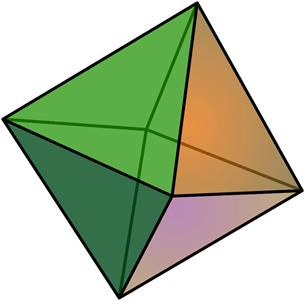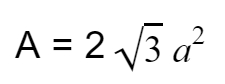# Program for Surface Area of Octahedron in C++

## What is Octahedron?

The word ‘dodecahedron’ is derived from the Greek words where, Octa means ‘Eight’ and hedron specifies ‘faces’. octahedron in geometric is a 3-D platonic or regular solid with eight faces. Like, other figures octahedrons also have properties and that are −

• 6 polyhedron vertices
• 12 polyhedron edges
• 8 equilateral faces

Given below is the figure of octahedron## Problem

Given with the sides, the program must find the surface area of octahedron where surface area is the total space occupied by the faces of the given figure.

To calculate surface area of octahedron there is a formula −Where, a is side of an Octahedron

## Example

Input-: side=5
Output-: 86.6025

## Algorithm

Start
Step 1 -> declare function to find area of octahedron
double surface_area(double side)
return (2*(sqrt(3))*(side*side))
Step 2 -> In main()
Declare variable double side=5
Print surface_area(side)
Stop

## Example

#include <bits/stdc++.h>
using namespace std;
//function for surface area of octahedron
double surface_area(double side){
return (2*(sqrt(3))*(side*side));
}
int main(){
double side = 5;
cout << "Surface area of octahedron is : " << surface_area(side);
}

## Output

Surface area of octahedron is : 86.6025